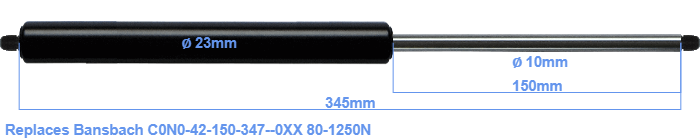# Replacement for Bansbach C0N0-42-150-347–0XX 80-1250N

\$30.83

Replacement gas spring for the Bansbach C0N0-42-150-347--0XX 80-1250 Newton. Thread: M8. Brand: HAHN, a Stabilus company.

Please note:
The diameter of the tube of this replacement gas spring is 23mm instead of 22mm.
 Force Choose an option80 Newton100 Newton120 Newton140 Newton150 Newton160 Newton180 Newton200 Newton220 Newton240 Newton250 Newton260 Newton280 Newton300 Newton320 Newton340 Newton350 Newton360 Newton380 Newton400 Newton420 Newton440 Newton450 Newton460 Newton480 Newton500 Newton520 Newton540 Newton550 Newton560 Newton580 Newton600 Newton620 Newton640 Newton650 Newton660 Newton680 Newton700 Newton720 Newton740 Newton750 Newton760 Newton780 Newton800 Newton820 Newton840 Newton850 Newton860 Newton880 Newton900 Newton920 Newton940 Newton950 Newton960 Newton980 Newton1000 Newton1020 Newton1040 Newton1050 Newton1060 Newton1080 Newton1100 Newton1120 Newton1140 Newton1150 Newton1160 Newton1180 Newton1200 Newton1220 Newton1240 Newton1250 NewtonClearThe diameter of the cylinder of this gas spring is 23 mm. The cross section of the rod is 10 mm. The rod (the chrome part) is 150 mm long. In total, the length is 345 millimeter. Keep in mind that this is the length between the centers of the mounting parts. The length from thread to thread (exlcuding the mounting parts) is 345 millimeter. This replacement gas spring has a force of 80-1250 Newton. Attention: this is not an official Bansbach gas spring, but a replacement gas spring. This replacement gas spring is a HAHN gas spring. The gas spring can be used without problems as a replacement, because the dimensions and force almost entirely equal.